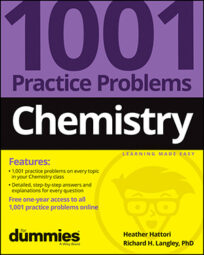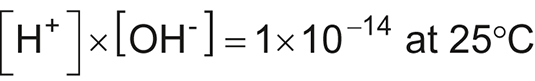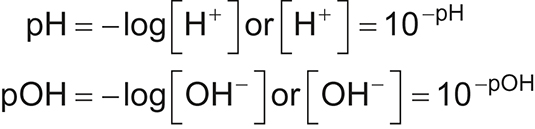##### Chemistry: 1001 Practice Problems For Dummies (+ Free Online Practice)Acid-base reactions and their associated calculations play a primary role in many chemical, biological, and environmental systems. Whether you’re determining hydrogen ion concentration, [H+]; hydroxide ion concentration, [OH˗]; pH; or pOH, an equation and a calculator are important tools to have in your toolbox. Following are some handy formulas for solving acid/base problems.

## Calculating hydrogen or hydroxide ion concentration

The following equation allows you to calculate the hydrogen ion concentration, [H+], at 25°C if you know the hydroxide ion concentration, [OH]; you can also find [OH] if you know [H+]. Just divide 1 × 1014 by the concentration given, and you get the concentration that you need. Tip: To use scientific notation on your calculator, use the EE or EXP key (followed by the exponent) rather than the × 10^ keys.## Calculating hydrogen or hydroxide ion concentration from the pH or pOH

Be familiar with how to solve for [H+] or [OH] when given the pH or pOH (or vice versa). Use the following formulas:Many scientific and graphing calculators differ in how they handle inputting values and taking logarithms, so know the proper keystroke order for your calculator. Be sure to review your calculator manual or look online.

## Calculating pH when given the pOH

Calculating pH when you know the pOH (or vice versa) is probably the easiest of the acid-base calculations. Here’s the formula:
pH + pOH = 14
Simply subtract the given value from 14 (keeping significant digits in mind) to get the value that you need.

## Doing titration calculations with a 1:1 acid-to-base ratio

When you’re given titration calculations where the acid and base are reacting in a 1:1 ratio according to the balanced equation, the following equation offers a quick and easy way to solve for either the concentration of one of the substances or the volume necessary to complete the titration:
MAVA = MBVB
If the acid and base aren’t reacting in a 1:1 ratio, use stoichiometry (or dimensional analysis) to solve for your unknown quantity. By the way, stoichiometry works for the 1:1 ratio questions, too; it just takes one or two more steps. Remember: Keep track of your units! Cancel what you need to get rid of and make sure that you still have the units you need in your final answer.